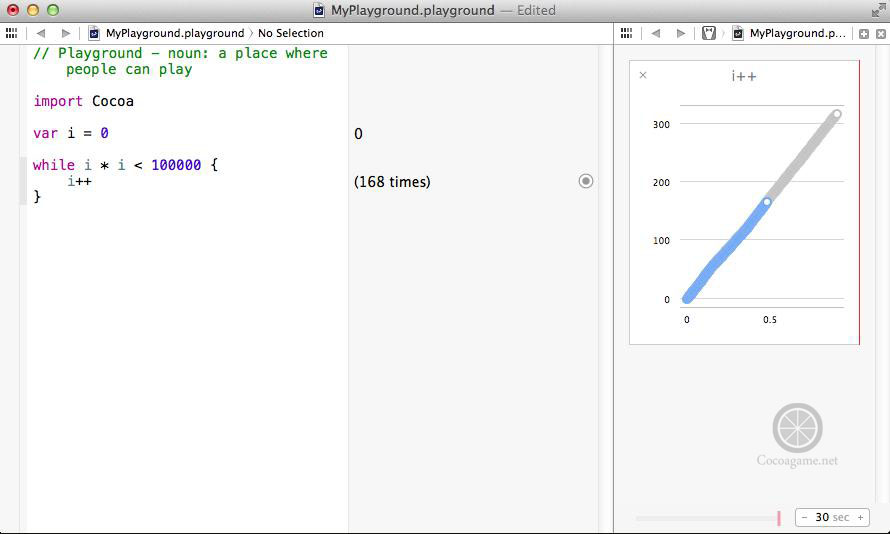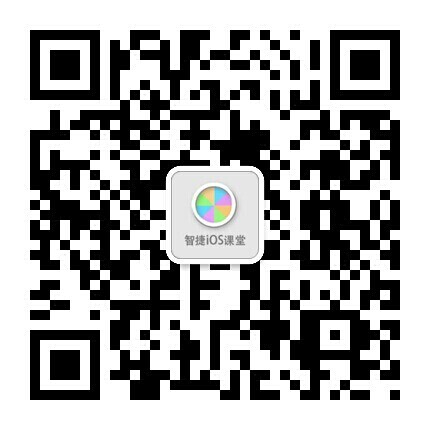# Swift中的循环语句

while语句是一种先判断的循环结构，格式如下：
while 循环条件 {
语句组
}
while循环没有初始化语句，循环次数是不可知的，只要循环条件满足，循环就会一直进行下去。

var i:Int64 = 0

while i * i < 100000 {
i++
}

println("i = \(i)")
println("i * i = \(i * i)")

i = 317
i * i = 100489

while true {
语句组
}do while语句的使用与while语句相似，不过do while语句是事后判断循环条件结构，语句格式如下：
do {
语句组
} while 循环条件
do while循环没有初始化语句，循环次数是不可知的，不管循环条件是否满足，都会先执行一次循环体，然后再判断循环条件。如果条件满足则执行循环体，不满足则停止循环。

var i:Int64 = 0

do{
i++
} while  i * i < 100000

println("i = \(i)")
println("i * i = \(i * i)")

i = 317
i * i = 100489

for语句是应用最广泛、功能最强的一种循环语句。一般格式如下：
for 初始化; 循环条件; 迭代 {
语句组
}

println("n   n*n")
println("---------")
for var i = 1; i < 10; i++  {
println("\(i) x \(i) = \(i * i)")
}

n   n*n
---------
1 x 1 = 1
2 x 2 = 4
3 x 3 = 9
4 x 4 = 16
5 x 5 = 25
6 x 6 = 36
7 x 7 = 49
8 x 8 = 64
9 x 9 = 81

for ; ;  {
......
}

var x:Int32
var y:Int32

for x = 0, y = 10; x < y; x++, y--  {
println("(x,y) = (\(x),\(y))")
}

(x,y) = (0,10)
(x,y) = (1,9)
(x,y) = (2,8)
(x,y) = (3,7)
(x,y) = (4,6)

Swift提供了一种专门用于遍历集合的for循环——for in循环。使用for in循环不必按照for的标准套路编写代码，只需要提供一个集合就可以遍历。

let numbers = [1, 2, 3, 4, 5, 6, 7, 8, 9, 10]

println("----for-------")
for var i = 0; i < countElements(numbers); i++ {
println("Count is: \(i)")
}

Count is: 0
Count is: 1
Count is: 2
Count is: 3
Count is: 4
Count is: 5
Count is: 6
Count is: 7
Count is: 8
Count is: 9

let numbers = [1, 2, 3, 4, 5, 6, 7, 8, 9, 10];

println("----for in----")
for item in numbers {
println("Count is: \(item)")
}

Count is: 0
Count is: 1
Count is: 2
Count is: 3
Count is: 4
Count is: 5
Count is: 6
Count is: 7
Count is: 8
Count is: 9©️2019 CSDN 皮肤主题: 大白 设计师: CSDN官方博客